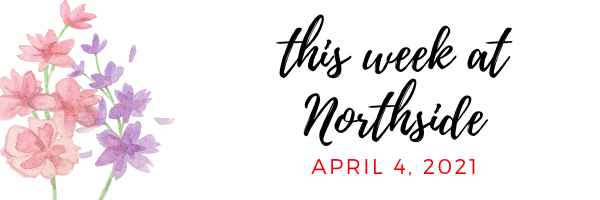/* styles */
 table div table+table+table+table div table{width:100%;padding:0}table div table+table+table+table div table img{width:96.23%;padding:0;float:none}table div table+table+table+table div table td{width:100%;padding:0 1.88% 18px}/* styles */# Upcoming Important Dates

Friday, April 9 - First Friday
Tuesday, April 20 - LSC Meeting
Friday, May 14 - Virtual Annual NCP Fundraiser

## News from the Counseling Office

▪ Class of 2021 Spring Checklist
▪ Chicago STEM Signing Day and Scholarship
▪ Apprenticeships
▪ Summer Enrichment/Pre-College Programs
▪ Chicago Teachers Union Scholarship
 ▪ Class of 2021 Spring Checklist
 ▪ Chicago STEM Signing Day and Scholarship
 ▪ Apprenticeships
 ▪ Summer Enrichment/Pre-College Programs
 ▪ Chicago Teachers Union Scholarship

## Sports, Yearbook & General School News

▪ LSC Update
▪ Pandemic Electronic Benefit Program
▪ Future Problem Solvers Coaching Opportunity
▪ Annual NCP Fundraiser - Save the Date
▪ Soccer Games This Week
▪ NCP Car Magnets and Window/Yard Signs Available
▪ Order Your 2020-2021 NCP Yearbook
▪ Ways for Parents to Stay in Touch/Volunteer/Support NCP/Social Media Links
 ▪ LSC Update
 ▪ Pandemic Electronic Benefit Program
 ▪ Future Problem Solvers Coaching Opportunity
 ▪ Annual NCP Fundraiser - Save the Date
 ▪ Soccer Games This Week
 ▪ NCP Car Magnets and Window/Yard Signs Available
 ▪ Order Your 2020-2021 NCP Yearbook
 ▪ Ways for Parents to Stay in Touch/Volunteer/Support NCP/Social Media Links
 table div table+table+table+table+table+table+table div table{width:100%;padding:0}table div table+table+table+table+table+table+table div table img{width:96.23%;padding:0;float:none}table div table+table+table+table+table+table+table div table td{width:100%;padding:0 1.88% 18px}/* styles */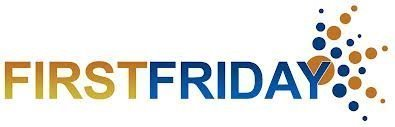# First Friday Virtual Gathering

Friday, April 9
9:00 am Zoom Meeting

Each month, NCP parents and guardians are invited to get together for a monthly coffee gathering. This month, join fellow NCP parents online. Stay for as long as your schedule allows.

Meeting ID: 848 7451 7349
Passcode: 336446
One tap mobile
+13126266799,,84874517349#,,,,336446# US (Chicago)
+19294362866,,84874517349#,,,,
336446# US (New York)

+1 312 626 6799 US (Chicago)

 table div table+table+table+table+table+table+table+table+table div table{width:100%;padding:0}table div table+table+table+table+table+table+table+table+table div table img{width:96.23%;padding:0;float:none}table div table+table+table+table+table+table+table+table+table div table td{width:100%;padding:0 1.88% 18px}/* styles */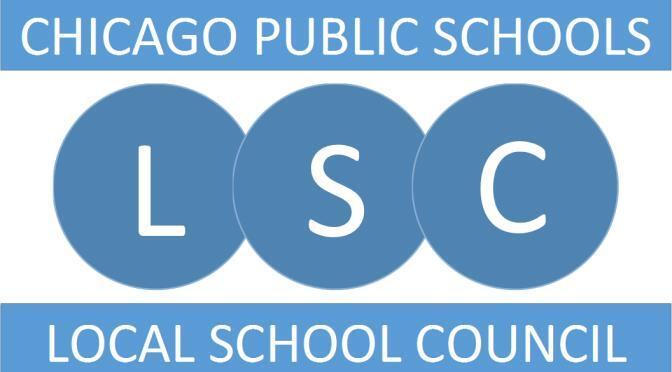# LSC Update

Dear NCP Community,

We want to thank everyone who participated in last week’s forums and/or surveys. We received great feedback that informed the position posting and will assist us as we move into the interview phase of the process.

We especially want to thank the students; 587 responded, 32% of them freshman.

HERE is the principal description that was posted on Wednesday. Feel free to share it with qualified candidates. The application deadline is Wednesday, April 7.

In the meantime, if you have any questions or comments, please email the LSC at northsidecollegepreplsc@gmail.com.

In addition, we have two new LSC members that we appointed at Tuesday’s meeting. Amanda Crowley is our new community representative. She is a teacher-librarian (see her candidate statement.)

In addition, Amy Hennessy is our new parent representative. She is the parent of a freshman and works for the U.S. Department of Health and Human Services (see her candidate statement.)

Our next regularly scheduled meeting is Tuesday, April 20 at 6:30 pm. We hope to see you there.

Sincerely,
~ NCP LSC - David Cihla, Amanda Crowley, Gregory DiFrancesco, Brad Hack, Amy Hennessy, Luna Johnston, John Lynch, Shari Massey, Marion McCreedy, Sandra Morales-Mirque, Martha Mulligan, Erika Schechter, and Patti Stuber

 table div table+table+table+table+table+table+table+table+table+table+table div table{width:100%;padding:0}table div table+table+table+table+table+table+table+table+table+table+table div table img{width:96.23%;padding:0;float:none}table div table+table+table+table+table+table+table+table+table+table+table div table td{width:100%;padding:0 1.88% 18px}/* styles */# Pandemic Electronic Benefit Program

All CPS students, regardless of income or citizenship, are eligible to receive food benefits as part of the US Government’s COVID-19 pandemic response. Funds will be loaded onto cards that will be mailed to each student at the primary address listed for them in ASPEN. To make sure your student receives the benefits check that your primary address is up to date in ASPEN.

Watch the mail for a plain envelope due to arrive this month. Be sure to keep the card because additional benefits will be loaded onto it going forward. For more information, check the March 3 post on SchoolCNXT.

 table div table+table+table+table+table+table+table+table+table+table+table+table+table div table{width:100%;padding:0}table div table+table+table+table+table+table+table+table+table+table+table+table+table div table img{width:96.23%;padding:0;float:none}table div table+table+table+table+table+table+table+table+table+table+table+table+table div table td{width:100%;padding:0 1.88% 18px}/* styles */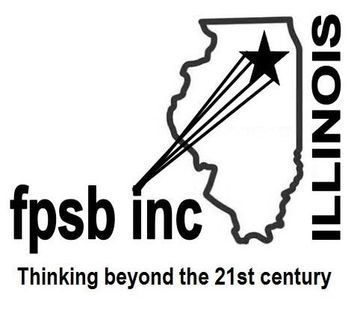# Future Problem Solvers Coaching Opportunity

The Future Problem Solvers Club needs a new coach for next year. FPS teams use the Six-Step Process to create an action plan to solve a problem identified in a scenario of a future situation. The teams of up to four students can compete in regional, state, and international level competitions.

Northside has typically fielded at least one team per grade. Individual competitions are also available. Whether students are interested in the arts and humanities or in STEM subjects, the Six-Step Process allows them to use their strengths in collaboration with other students as they identify challenges and articulate underlying problems, brainstorm possible solutions, generate criteria to evaluate those solutions, and then come up with an action plan to address the problem.

Training is required and provided by FPS Illinois. For more information, contact Janice Cody at jcody6933@gmail.com.

 table div table+table+table+table+table+table+table+table+table+table+table+table+table+table+table div table{width:100%;padding:0}table div table+table+table+table+table+table+table+table+table+table+table+table+table+table+table div table img{width:96.23%;padding:0;float:none}table div table+table+table+table+table+table+table+table+table+table+table+table+table+table+table div table td{width:100%;padding:0 1.88% 18px}/* styles */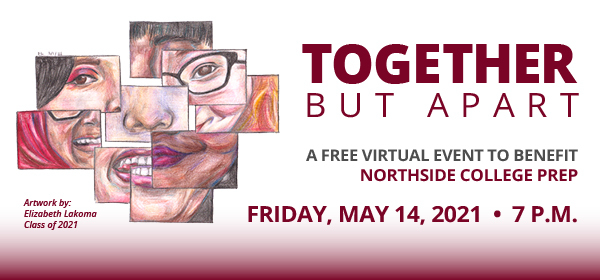# Save the date for the annual Northside College Prep Fundraiser

## Friday, May 14, 7:00 pm

Please save the date for the annual Northside College Prep fundraiser. on May 14 at 7:00 pm. While we can't be together this year to celebrate, we can be "Together but Apart," which is the theme for this year's virtual benefit! We hope you can join us in a fun virtual program showcasing the student talent within our community. Tell your friends, family, and grandparents to join!

And while we have you, please consider helping us with items and experiences for our fundraiser silent auction. We need your help! Start brainstorming something that our families would love, whether it be a local business gift card, time in a vacation home, or another hot item! In addition, if you have a business or company that would make for a good corporate sponsor for the benefit, we would love to have you! Reach out to Linda Weil at Lindaweil-silk@hotmail.com by Sunday, April 25 with ideas for the auction and for corporate sponsorship.

We can't wait to celebrate "together" soon!

 table div table+table+table+table+table+table+table+table+table+table+table+table+table+table+table+table+table+table div table{width:100%;padding:0}table div table+table+table+table+table+table+table+table+table+table+table+table+table+table+table+table+table+table div table img{width:96.23%;padding:0;float:none}table div table+table+table+table+table+table+table+table+table+table+table+table+table+table+table+table+table+table div table td{width:100%;padding:0 1.88% 18px}/* styles */# Class of 2021: Spring Checklist

Class of 2021 Parents,

The end of the school year is rapidly approaching. With all of the duties and responsibilities your student is expected to complete between now and June, the counseling department would like to encourage your family to discuss the following checklists and create a plan to address the relevant action items.

Consider completing the following action items on the Class of 2021: Check-out List (click here) as well as the additional action items listed on the Spring Checklist (click here) to assist you with remaining on track for the second semester.

If you would like to schedule a one on one with Ms. Moore via Calendly, visit https://calendly.com/mcmoore6/ or contact her via e-mail at mcmoore6@cps.edu. Appointments are available during lunch blocks, after school, and during parent-teacher conferences in April.

 table div table+table+table+table+table+table+table+table+table+table+table+table+table+table+table+table+table+table+table+table div table{width:100%;padding:0}table div table+table+table+table+table+table+table+table+table+table+table+table+table+table+table+table+table+table+table+table div table img{width:96.23%;padding:0;float:none}table div table+table+table+table+table+table+table+table+table+table+table+table+table+table+table+table+table+table+table+table div table td{width:100%;padding:0 1.88% 18px}/* styles */# Chicago STEM Signing Day and Scholarship

All Chicago Public School (CPS) graduating high school seniors who will be pursuing a STEM degree in a post-secondary school in 2021 are eligible to apply. Students must submit an online application detailing their academic and after-school experiences, personal goals, and aspirations to pursue a STEM degree and career pathway.

Honorees are awarded a scholarship and placement with mentors, and join a community of trailblazers in STEM.

To learn more about the 2021 application process and access the online form please visit: www.chicagostempathways.org/ssd-chicago. Also view the English or Spanish flyers for more details.

## What is STEM Signing Day?

Just like student-athletes who sign letters of intent with their chosen schools, STEM Signing Day celebrates outstanding students who have made a commitment to pursue a STEM degree.

## Eligibility Criteria

▪ Graduating senior at a CPS high school;
▪ Attending college in 2021;
▪ Pursuing a STEM degree;
▪ Get support from your teacher, guidance counselor, after-school program facilitator, etc.
 ▪ Graduating senior at a CPS high school;
 ▪ Attending college in 2021;
 ▪ Pursuing a STEM degree;
 ▪ Get support from your teacher, guidance counselor, after-school program facilitator, etc.

## Honorees

▪ Earn a scholarship;
▪ Receive recognition at STEM Signing Day event;
▪ Join a community of students, STEM professionals, and mentors
 ▪ Earn a scholarship;
 ▪ Receive recognition at STEM Signing Day event;
 ▪ Join a community of students, STEM professionals, and mentors

Applications are due April 9, 2021.

 table div table+table+table+table+table+table+table+table+table+table+table+table+table+table+table+table+table+table+table+table+table+table div table{width:100%;padding:0}table div table+table+table+table+table+table+table+table+table+table+table+table+table+table+table+table+table+table+table+table+table+table div table img{width:96.23%;padding:0;float:none}table div table+table+table+table+table+table+table+table+table+table+table+table+table+table+table+table+table+table+table+table+table+table div table td{width:100%;padding:0 1.88% 18px}/* styles */# Apprenticeships

The Apprenticeship Pathway involves getting paid as you learn a highly skilled profession. You’ll get an inside look at what life is really like in a certain industry or at a specific company and earn a living wage. Different industries offer apprenticeship programs, including:
▪ Construction
▪ Manufacturing
▪ Health care
▪ Information technology
▪ Transportation
▪ Energy
▪ Other

If an apprenticeship is a postsecondary option that interests you, consider taking the next steps:

## Is an apprenticeship the right plan for you?

▪ Meet with your counselor, teachers, family members, and other advisors to get help as you explore if an apprenticeship is right for you.
 ▪ Meet with your counselor, teachers, family members, and other advisors to get help as you explore if an apprenticeship is right for you.

## Explore apprenticeships

▪ Search for opportunities using Apprenticeship Finder or the Illinois Department of Employment Security (IDES) website. You can also search under Apprenticeship "related links” at cps.edu/LearnPlanSucceed.
 ▪ Search for opportunities using Apprenticeship Finder or the Illinois Department of Employment Security (IDES) website. You can also search under Apprenticeship "related links” at cps.edu/LearnPlanSucceed.

## Apply for Apprenticeship Opportunities

▪ The City Colleges of Chicago website offers apprenticeship programs that can take you from student to employee, opening the door into several of Chicago’s leading businesses.
 ▪ The City Colleges of Chicago website offers apprenticeship programs that can take you from student to employee, opening the door into several of Chicago’s leading businesses.
 table div table+table+table+table+table+table+table+table+table+table+table+table+table+table+table+table+table+table+table+table+table+table+table+table div table{width:100%;padding:0}table div table+table+table+table+table+table+table+table+table+table+table+table+table+table+table+table+table+table+table+table+table+table+table+table div table img{width:96.23%;padding:0;float:none}table div table+table+table+table+table+table+table+table+table+table+table+table+table+table+table+table+table+table+table+table+table+table+table+table div table td{width:100%;padding:0 1.88% 18px}/* styles */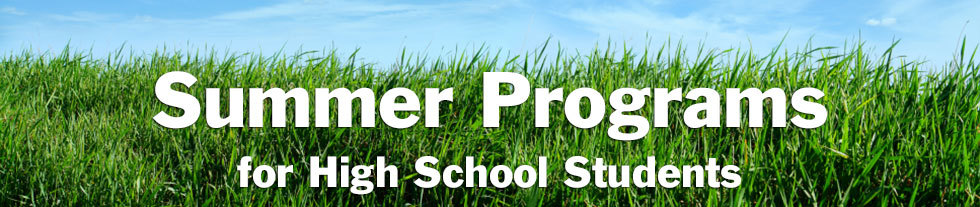# Summer Enrichment/Pre-College Programs

In addition to the virtual information sessions Northside Prep is offering after school daily and during the school day on Wednesdays (click HERE), the counseling department would like to share additional information about information sessions for summer enrichment opportunities.

Are you and your student interested in learning about enrichment opportunities or pre-college programs to engage in during the spring or summer? Would you like to build your resume and enhance your academic, personal, or social skills? If the answer is yes, consider reviewing the following resources to assist your family with identifying the best fit enrichment opportunities for your student:

 table div table+table+table+table+table+table+table+table+table+table+table+table+table+table+table+table+table+table+table+table+table+table+table+table+table+table+table div table{width:100%;padding:0}table div table+table+table+table+table+table+table+table+table+table+table+table+table+table+table+table+table+table+table+table+table+table+table+table+table+table+table div table img{width:96.23%;padding:0;float:none}table div table+table+table+table+table+table+table+table+table+table+table+table+table+table+table+table+table+table+table+table+table+table+table+table+table+table+table div table td{width:100%;padding:0 1.88% 18px}/* styles */# Chicago Teachers Union Scholarship

The Chicago Teachers Union Scholarship Committee is working hard to adapt and maintain our annual tradition of awarding scholarships to children of our members who are graduating and have plans to pursue post-secondary education. If you have a child who will be graduating from high school this year, NOW is the time for them to apply for a CTU/CTUF Scholarship! The deadline is May 10.

Scholarships in the amount of \$1,000 will be awarded to students to help defray the cost of post-secondary education in college or vocational programs. Details are available at www.ctulocal1.org/scholarship.

## Eligibility

Awards are entirely unrestricted as to race, religion, political affiliation, or choice of an accredited college, university, business, trade, or vocational school.

The applicant must be a child of a currently employed, active member in good standing of the Chicago Teachers Union, or a child of a deceased member who was in good standing with the Chicago Teachers Union at the time of death. They must also be a high school senior who will graduate this year before August 31. Special education students are eligible for all scholarships and are encouraged to apply.

## How to Apply

Please make certain that the application is completed in full, and that it is signed by the applicant AND the parent or guardian so that membership in good standing can be verified before presentation to the Scholarship Committee of the Chicago Teachers Union.

## Application Info

Scholarship winners will be notified by mail or email and invited to attend a special virtual reception and award ceremony with their families.

 table div table+table+table+table+table+table+table+table+table+table+table+table+table+table+table+table+table+table+table+table+table+table+table+table+table+table+table+table+table div table{width:100%;padding:0}table div table+table+table+table+table+table+table+table+table+table+table+table+table+table+table+table+table+table+table+table+table+table+table+table+table+table+table+table+table div table img{width:96.23%;padding:0;float:none}table div table+table+table+table+table+table+table+table+table+table+table+table+table+table+table+table+table+table+table+table+table+table+table+table+table+table+table+table+table div table td{width:100%;padding:0 1.88% 18px}/* styles */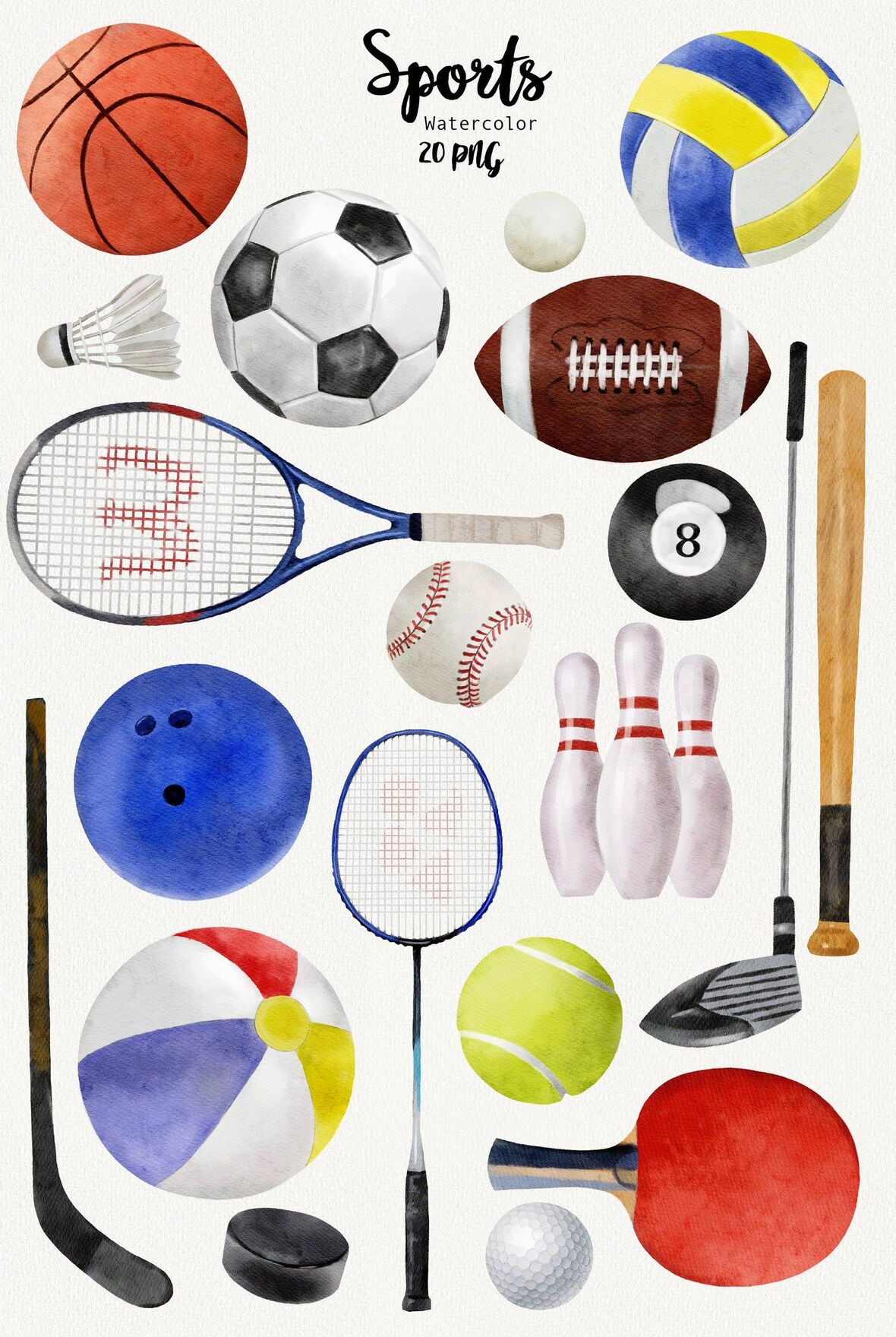# Soccer Games This Week

Boys Soccer
▪ Monday, April 5 vs. Mather @ Winnemac Stadium (V at 4:30 pm)
▪ Saturday, April 10 vs. Notre Dame Niles @ Notre Dame Niles (JV at 1:00 pm) vs. Notre Dame College Prep @ Notre Dame College Prep (V at 3:00 pm)

The athletic department is looking for volunteers to help live stream our volleyball matches and possibly other athletic events. Anyone interested in volunteering should contact athletic director Henry Henderson at hhenderson@cps.edu.

 table div table+table+table+table+table+table+table+table+table+table+table+table+table+table+table+table+table+table+table+table+table+table+table+table+table+table+table+table+table+table+table div table{width:100%;padding:0}table div table+table+table+table+table+table+table+table+table+table+table+table+table+table+table+table+table+table+table+table+table+table+table+table+table+table+table+table+table+table+table div table img{width:96.23%;padding:0;float:none}table div table+table+table+table+table+table+table+table+table+table+table+table+table+table+table+table+table+table+table+table+table+table+table+table+table+table+table+table+table+table+table div table td{width:100%;padding:0 1.88% 18px}/* styles */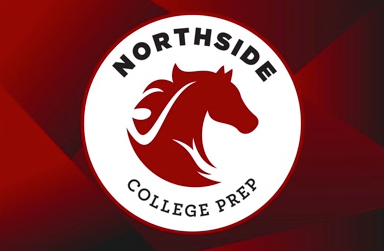# NCP Car Magnets and Window/Yard Signs Available

Friends of Northside is pleased to offer NCP car magnets for sale and to reopen orders for NCP yard/window signs. Show your school pride!

If you are interested, please click the button below. Each time we reach minimums for each item we will send for production and notify you of pickup timing. We anticipate two print runs for magnets and at least one for signs this school year. Go Mustangs!

Other swag is made available via the Saddle Shop directly via NCP; timing varies for their online pop-ups.

 table div table+table+table+table+table+table+table+table+table+table+table+table+table+table+table+table+table+table+table+table+table+table+table+table+table+table+table+table+table+table+table+table+table+table div table{width:100%;padding:0}table div table+table+table+table+table+table+table+table+table+table+table+table+table+table+table+table+table+table+table+table+table+table+table+table+table+table+table+table+table+table+table+table+table+table div table img{width:96.23%;padding:0;float:none}table div table+table+table+table+table+table+table+table+table+table+table+table+table+table+table+table+table+table+table+table+table+table+table+table+table+table+table+table+table+table+table+table+table+table div table td{width:100%;padding:0 1.88% 18px}/* styles */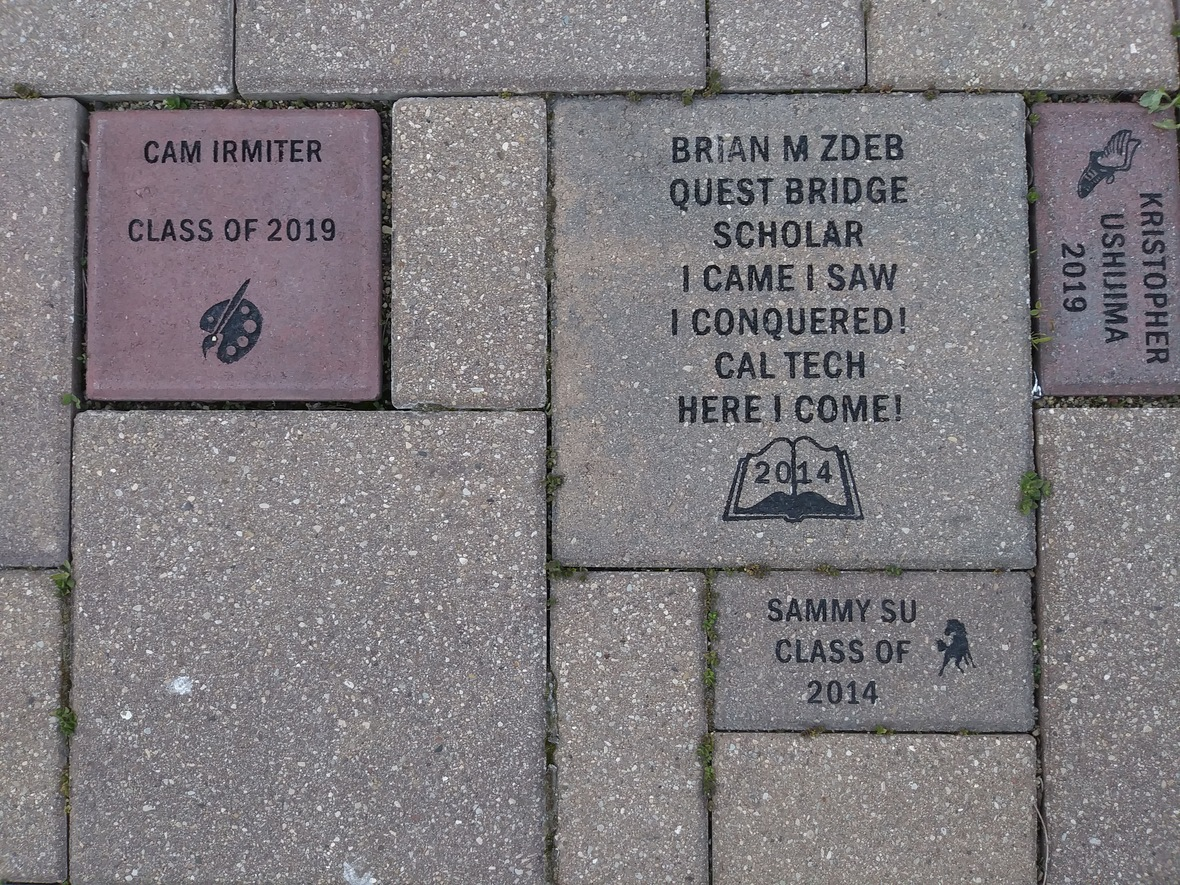Consider buying a custom NCP Brick for your child this year. Your brick, engraved with a personal message, will become a permanent part of the Northside College Prep Celestial Garden Walkway.

Your brick may be purchased in three sizes:

▪ 4" x 8" = \$50
▪ 8" x 8" = \$100
▪ 12" x 12" = \$150

Friends of Northside will provide a gift certificate acknowledging the purchase. Janet Jasmer will assist with the process and can answer any questions at jjasmer@atproperties.com.

 table div table+table+table+table+table+table+table+table+table+table+table+table+table+table+table+table+table+table+table+table+table+table+table+table+table+table+table+table+table+table+table+table+table+table+table+table div table{width:100%;padding:0}table div table+table+table+table+table+table+table+table+table+table+table+table+table+table+table+table+table+table+table+table+table+table+table+table+table+table+table+table+table+table+table+table+table+table+table+table div table img{width:96.23%;padding:0;float:none}table div table+table+table+table+table+table+table+table+table+table+table+table+table+table+table+table+table+table+table+table+table+table+table+table+table+table+table+table+table+table+table+table+table+table+table+table div table td{width:100%;padding:0 1.88% 18px}/* styles */# Any Questions About NCP Communications Sent Via CNXT?

If you are having problems with SchoolCNXT, please contact the main office from 7:30 am - 3:15 pm at 773-534-3954, or you can email the NCP school clerk, Mary Grasz, at mtgrasz@cpu.edu.

NCP uses SchoolCNXT to communicate important information to parents and the school community. All families should have received a text message and/or email communication about signing up. If you did not receive that communication or need access to your student's account, you can gain access by using this tool on Northside's website.

 table div table+table+table+table+table+table+table+table+table+table+table+table+table+table+table+table+table+table+table+table+table+table+table+table+table+table+table+table+table+table+table+table+table+table+table+table+table+table+table div table{width:100%;padding:0}table div table+table+table+table+table+table+table+table+table+table+table+table+table+table+table+table+table+table+table+table+table+table+table+table+table+table+table+table+table+table+table+table+table+table+table+table+table+table+table div table img{width:96.23%;padding:0;float:none}table div table+table+table+table+table+table+table+table+table+table+table+table+table+table+table+table+table+table+table+table+table+table+table+table+table+table+table+table+table+table+table+table+table+table+table+table+table+table+table div table td{width:100%;padding:0 1.88% 18px}/* styles */table.module-39{width:68.87%;padding:0}table div table+table+table+table+table+table+table+table+table+table+table+table+table+table+table+table+table+table+table+table+table+table+table+table+table+table+table+table+table+table+table+table+table+table+table+table+table+table+table+table div table{width:68.87%;float:none;margin-left:auto;margin-right:auto;padding:0}table div table+table+table+table+table+table+table+table+table+table+table+table+table+table+table+table+table+table+table+table+table+table+table+table+table+table+table+table+table+table+table+table+table+table+table+table+table+table+table+table div table a{border:0 none;text-decoration:none}table div table+table+table+table+table+table+table+table+table+table+table+table+table+table+table+table+table+table+table+table+table+table+table+table+table+table+table+table+table+table+table+table+table+table+table+table+table+table+table+table div table img{width:100%!important;border:0 none;text-decoration:none}table div table+table+table+table+table+table+table+table+table+table+table+table+table+table+table+table+table+table+table+table+table+table+table+table+table+table+table+table+table+table+table+table+table+table+table+table+table+table+table+table div table td{width:100%;padding:0}/* styles */

# Order a Yearbook Today!

Interested in purchasing a copy of the 2020-2021 Stampede, NCP's yearbook? Click HERE to start your order! And if you didn't get a chance to purchase a copy of the 2019-2020 yearbook, you now have a change to buy one of those, too! Just email Ms. McCreedy at mkmccreedy@cps.edu after you've completed your 2020-2021 order.

Copies are now \$55.

 table div table+table+table+table+table+table+table+table+table+table+table+table+table+table+table+table+table+table+table+table+table+table+table+table+table+table+table+table+table+table+table+table+table+table+table+table+table+table+table+table+table+table+table div table{width:100%;padding:0}table div table+table+table+table+table+table+table+table+table+table+table+table+table+table+table+table+table+table+table+table+table+table+table+table+table+table+table+table+table+table+table+table+table+table+table+table+table+table+table+table+table+table+table div table img{width:96.23%;padding:0;float:none}table div table+table+table+table+table+table+table+table+table+table+table+table+table+table+table+table+table+table+table+table+table+table+table+table+table+table+table+table+table+table+table+table+table+table+table+table+table+table+table+table+table+table+table div table td{width:100%;padding:0 1.88% 18px}/* styles */table div table+table+table+table+table+table+table+table+table+table+table+table+table+table+table+table+table+table+table+table+table+table+table+table+table+table+table+table+table+table+table+table+table+table+table+table+table+table+table+table+table+table+table+table+table div table{width:100%;padding:0}table div table+table+table+table+table+table+table+table+table+table+table+table+table+table+table+table+table+table+table+table+table+table+table+table+table+table+table+table+table+table+table+table+table+table+table+table+table+table+table+table+table+table+table+table+table div table img{width:96.23%;padding:0;float:none}table div table+table+table+table+table+table+table+table+table+table+table+table+table+table+table+table+table+table+table+table+table+table+table+table+table+table+table+table+table+table+table+table+table+table+table+table+table+table+table+table+table+table+table+table+table div table td{width:100%;padding:0 1.88% 18px}/* styles */# Do you have news or team results to share with the Northside parent community?

 table div table+table+table+table+table+table+table+table+table+table+table+table+table+table+table+table+table+table+table+table+table+table+table+table+table+table+table+table+table+table+table+table+table+table+table+table+table+table+table+table+table+table+table+table+table+table+table div table{width:100%;padding:0}table div table+table+table+table+table+table+table+table+table+table+table+table+table+table+table+table+table+table+table+table+table+table+table+table+table+table+table+table+table+table+table+table+table+table+table+table+table+table+table+table+table+table+table+table+table+table+table div table img{width:96.23%;padding:0;float:none}table div table+table+table+table+table+table+table+table+table+table+table+table+table+table+table+table+table+table+table+table+table+table+table+table+table+table+table+table+table+table+table+table+table+table+table+table+table+table+table+table+table+table+table+table+table+table+table div table td{width:100%;padding:0 1.88% 18px}/* styles */# Support Northside - Shop AmazonSmile

AmazonSmile is a simple and automatic way to support Northside every time you shop.

Please note that our AmazonSmile name is Friends of "North" "Side" (North Side - two words at AmazonSmile).

 table div table+table+table+table+table+table+table+table+table+table+table+table+table+table+table+table+table+table+table+table+table+table+table+table+table+table+table+table+table+table+table+table+table+table+table+table+table+table+table+table+table+table+table+table+table+table+table+table+table div table{width:100%;padding:0}table div table+table+table+table+table+table+table+table+table+table+table+table+table+table+table+table+table+table+table+table+table+table+table+table+table+table+table+table+table+table+table+table+table+table+table+table+table+table+table+table+table+table+table+table+table+table+table+table+table div table img{width:96.23%;padding:0;float:none}table div table+table+table+table+table+table+table+table+table+table+table+table+table+table+table+table+table+table+table+table+table+table+table+table+table+table+table+table+table+table+table+table+table+table+table+table+table+table+table+table+table+table+table+table+table+table+table+table+table div table td{width:100%;padding:0 1.88% 18px}/* styles */# Follow Us on Social Media

 table div table+table+table+table+table+table+table+table+table+table+table+table+table+table+table+table+table+table+table+table+table+table+table+table+table+table+table+table+table+table+table+table+table+table+table+table+table+table+table+table+table+table+table+table+table+table+table+table+table+table+table div table{width:100%;padding:0}table div table+table+table+table+table+table+table+table+table+table+table+table+table+table+table+table+table+table+table+table+table+table+table+table+table+table+table+table+table+table+table+table+table+table+table+table+table+table+table+table+table+table+table+table+table+table+table+table+table+table+table div table img{width:96.23%;padding:0;float:none}table div table+table+table+table+table+table+table+table+table+table+table+table+table+table+table+table+table+table+table+table+table+table+table+table+table+table+table+table+table+table+table+table+table+table+table+table+table+table+table+table+table+table+table+table+table+table+table+table+table+table+table div table td{width:100%;padding:0 1.88% 18px}/* styles */table div table+table+table+table+table+table+table+table+table+table+table+table+table+table+table+table+table+table+table+table+table+table+table+table+table+table+table+table+table+table+table+table+table+table+table+table+table+table+table+table+table+table+table+table+table+table+table+table+table+table+table+table+table div table{width:100%;padding:0}table div table+table+table+table+table+table+table+table+table+table+table+table+table+table+table+table+table+table+table+table+table+table+table+table+table+table+table+table+table+table+table+table+table+table+table+table+table+table+table+table+table+table+table+table+table+table+table+table+table+table+table+table+table div table img{width:96.23%;padding:0;float:none}table div table+table+table+table+table+table+table+table+table+table+table+table+table+table+table+table+table+table+table+table+table+table+table+table+table+table+table+table+table+table+table+table+table+table+table+table+table+table+table+table+table+table+table+table+table+table+table+table+table+table+table+table+table div table td{width:100%;padding:0 1.88% 18px}/* styles */table div table+table+table+table+table+table+table+table+table+table+table+table+table+table+table+table+table+table+table+table+table+table+table+table+table+table+table+table+table+table+table+table+table+table+table+table+table+table+table+table+table+table+table+table+table+table+table+table+table+table+table+table+table+table+table div table{width:100%;padding:0}table div table+table+table+table+table+table+table+table+table+table+table+table+table+table+table+table+table+table+table+table+table+table+table+table+table+table+table+table+table+table+table+table+table+table+table+table+table+table+table+table+table+table+table+table+table+table+table+table+table+table+table+table+table+table+table div table img{width:96.23%;padding:0;float:none}table div table+table+table+table+table+table+table+table+table+table+table+table+table+table+table+table+table+table+table+table+table+table+table+table+table+table+table+table+table+table+table+table+table+table+table+table+table+table+table+table+table+table+table+table+table+table+table+table+table+table+table+table+table+table+table div table td{width:100%;padding:0 1.88% 18px}/* styles */